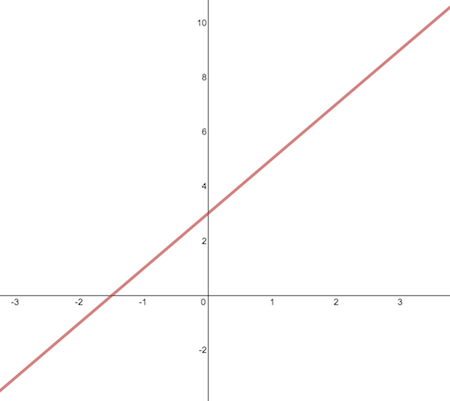Calculus

Slope of a CurveSally drew the line $y=2x+3$ on the board. What is the slope of this line?

What is the slope of the line tangent to the curve $y = \ln x + 20x$ at $x = \frac{1}{7}$ ?

What is the slope of the line tangent to the graph of the function $f(x) = - \frac{4}{x}$ at $x = \frac{1}{9}$ ?

What is the slope of the line tangent to the graph of the function $f(x) = 6 \cos x + 6 \sin x$ at $x = 20 \pi$ ?

What is the slope of the tangent line of the curve $f(x) = x^2 + 2013$ at $x = 29$?

×